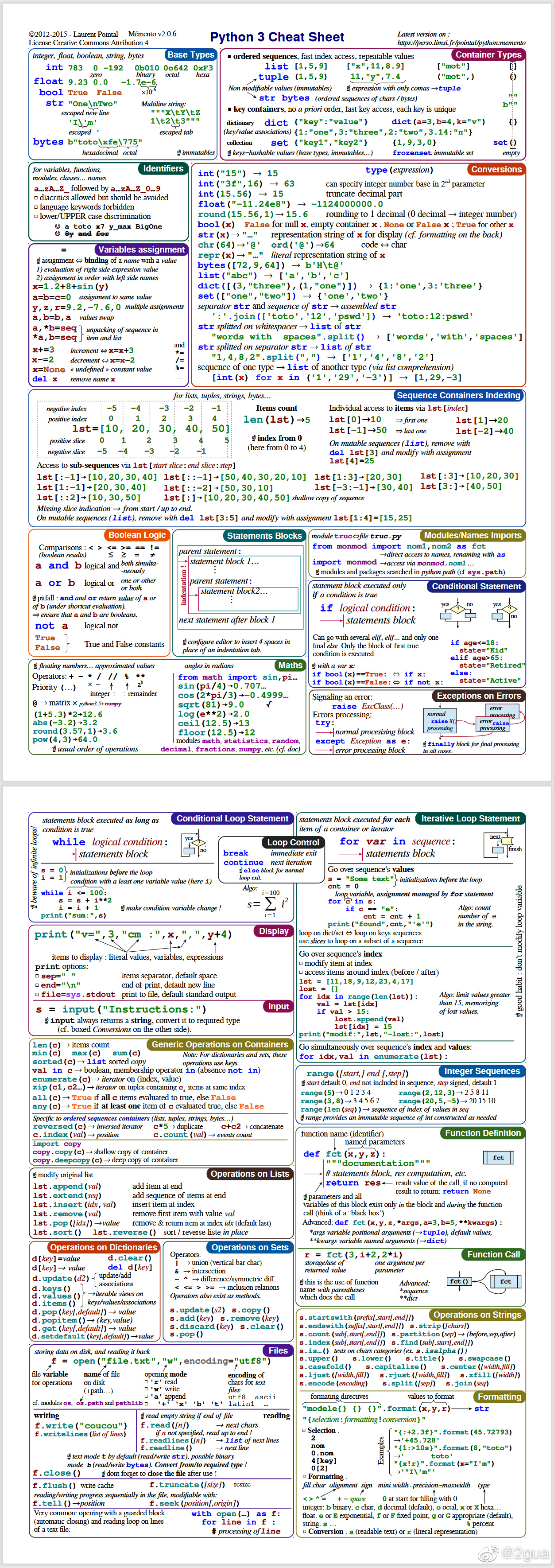## Crossin编程学习+高淇老师

• 注先前的Fn键，笔记本锁定了，可以用Fn+ESC恢复原有的功能。
• pycharm下，运行：Shift + F10
• 自动补全前面曾经出现过的单词： Alt+/
• 调出帮助文档：选中然后shift  F1
• 一句内容过长，使用行连接符\
• pycharm 代码，选定任何一行，代码栏下面(灰色的一小栏)会显示控制流结构，很重要

• 缩进决定逻辑层次
•  避免 tab 与空格混合的缩进风格，虽然我们知道大多数编辑器默认 tab 制表符就是 4 个空格

## 数字/布尔/字符串

### 整数和浮点数

• 数据类型转换(labview中也经常用到)：
• int(x) 或 float(x) 将x转换成整数或者浮点数
• str(x)可以将一个数字转换为字符串
• 进制转化，比如int("0xff",16)得到的就是十进制下的255，其中16表示0xff是16进制
如果要将16进制的0xff转换为2进制，那么还是得用十进制作为过渡，bin(int("0xff",16))，得到0b11111111

• 增强型赋值运算：我们常用 a += 2表示a = a+2，需要特别注意的是+=中间没有空格，运算符+、-、*，/、//、**和%和赋值符=结合可以构成增强型赋值运算符。
• 除法和幂次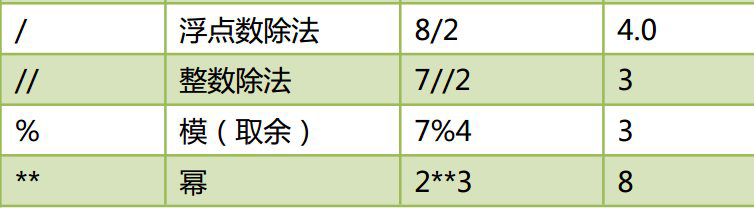• pow(2, 3) = 8也可以表示幂运算；
• divmod(13, 3)得到的是一个元组(4, 1)前者是商，后者是余数；

range的使用

• range 对象是一个迭代器对象，用来产生指定范围的数字序列。格式为：
range(start, end, [step])
• for i in range(10) 产生序列：0 1 2 3 4 5 6 7 8 9
• for i in range(3,10) 产生序列：3 4 5 6 7 8 9
• for i in range(3,10,2) 产生序列：3 5 7 9   #最后一个2表示步长

### 布尔

【同一运算符/相等运算符】：

• is for reference equality. It's used to know if two references refer (or point) to the same object, i.e if they're identical. Two objects are identical if they have the same memory address. 其实就是看id(object)是否相等。
• == is for value equality. It's used to know if two objects have the same value. 默认调用对象的__eq__()方法。
• is 运算符比 == 效率高，在变量和 None 进行比较时，应该使用 is。

【逻辑运算符】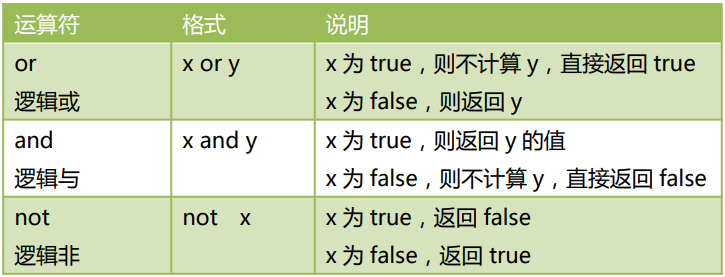bool类型转换，转成bool类型，下列情况被认为是False：

• 为0的数字，包括0，0.0，比如bool(0) == False
• 空字符串，包括”，””
• 表示空值的 None
• 空集合，包括()，[]，{}
• 在 if、while 等条件判断语句里，判断条件会自动进行一次bool的转换。比如下面的等价表达
a = '123'
if a:
print ('this is not a blank string')

if bool(a) == True:

if a != '':

• 如果赋值a = False，那么print(a)的结果是False，print(a==False)的结果是True；
• value = 2 < 5那么print(value)得到True；
•  bool and a or b —— 当bool条件为真时，结果是a；当bool条件为假时，结果是b，这其实就是labview中的select function。and-or似乎只是替代if-else而已，其实不然，在特殊场合，不能用if语句，这时就只能选择and-or技巧了，比如lambda函数中(后面会详述)；
• print ((a > 0) and “big” or “small”)的意思就是如果a >0成立就输入big，否则输出small；
• 特殊的情况说明：对于print ((a > 0) and “big” or “small”)，当a本身是个假值（如0，””）时，会出现误判，即使前面的bool是真，程序仍然选择输出b。确保a的值不会为假。最常用的方式是使 a 成为 [a] 、 b 成为 [b]，然后使用返回值列表的第一个元素：
a = ""
b = "hell"
c = (True and [a] or [b])
print(c)

a = True
print(a or 20/0)
# the output is "True"

• 成员资格操作符，in /not in 关键字，判断某个字符(子字符串)是否存在于某个list或者字符串中

a=["a","b","c"]   #这是对list
>>>"a" in a
True
>>>"a" not in a
True
>>>"a" in “abc”   #这是对单个字符串
True


### 字符串-基本操作

(和list的用法类似，有些用法就不在list里面赘述)

• 遍历
word = 'helloworld'
for c in word:
print(c)
• 索引(与list不同的是，字符串不能通过索引访问去更改其中的字符)
print (word)
print (word[-2])  # 倒数第二个字符
• 切片     # [起始偏移量 start：终止偏移量 end：步长 step]
print (word[5:7])
print (word[:-5])
print (word[:])
• 连接join
a = ','.join('word')
print(a)得到w,o,r,d
• 分割split()
• sentence = 'I am an English sentence'
a=sentence.split()   （默认按照空格/换行/制表符分割）
•   指定分隔符.
section = 'Hi. I am the one. Bye.'
a=section.split('.')
得到['Hi', ' I am the one', ' Bye', '']
• 大小写转换

s = What Is Your Name?
print(s.swapcase()) #大小写互换

s.lower()-变成小写
s.upper()-变成大写
s.swapcase()-大小写互换
a.capitalize()-产生新的字符串,首字母 大写
a.title()-产生新的字符串,每个单 词都首字母大写

• 去除首尾特定字符串——strip()函数 (remove函数是用于列表的，不能直接用于字符串。)
• .lstrip()   去除左边的
• .rstrip()   去除右边的
• .strip()    去除左右两边的
例子：可以用于移除空格，比如
>>>t.strip( )    #去掉左右空格，其实可以写 strip() ，一样功能

• len(a)    字符串的长度
• a.startswith("xxxxx") 以指定字符串开头，给出布尔值
• a.endswith("xxx")以指定字符串结尾，给出布尔值
• a.find("xx")   第一次出现特定字符串的位置     #如果找不到，返回-1
• a.index("xx")  第一次出现特定字符串的位置    #如果找不到，抛出异常
• a.rfind("xx")   最后一次出现特定字符串的位置
• a.count("xx")  指定字符串出现的次数
• a.isalnum()    所有字符全是数字或字母，给出布尔值

• "3"+"2" ==> "32"
• 元组和列表也可以，比如[10,20,30]+[5,10,100] ==>[10,20,30,5,10,100]
• "txt"*3 ==> "txttxttxt"
• 元组和列表也可以，比如[10,20,30]*3 ==> [10,20,30,10,20,30,10,20,30]

• python3直接支持Unicode(16位，二进制)，可以表示世界上任何书面语言的字符( LabVIEW 没有完全支持 unicode)，Python3的字符默认就是16位Unicode编码，ASCII码(8位，只够英文)是Unicode编码的子集；
• 使用内置函数 ord() 可以把字符转换成对应的Unicode码，比如ord("A")得到65，ord("高")得到39640；
• 使用内置函数 chr() 的可以把十进制数字转换成对应的字符，比如chr(66)得到'B'；

【可变字符串】：一般来说，字符串创建之后是不可变的，但是如果真的要频繁修改某个字符串(在原地址上修改)，那么可以使用 io.StringIO对象或 array 模块 。具体看高老师的资料，这里不赘述。(感觉用到的概率不大)

### 字符串-正则表达式

(1)  最简单的正则表达式，只有基本的字母或数字，它满足的匹配规则就是完全匹配

import re
text = "Hi, I am Shirley Hilton. I am his wife."
m =  re.findall( r"hi", text)
if m:
print (m)
else:
print ('not match')

# output is ['hi', 'hi']

\b表示字母数字与非字母数字的边界， 非字母数字与字母数字的边界。

“*”表示任意数量连续字符

r"\bhi"表示不对""内的字符串进行转义。\有转义的功能，比如\n表示换行。r是raw的意思，表示按照原始的模样。

import re的re就是python的正则表达式模块(库)，findall是其中一个方法，用来按照提供的正则表达式，去匹配文本中的所有符合条件的字符串。返回结果是一个包含所有匹配的list。

“\S”，它表示的是不是空白符的任意字符(一个)。匹配结果为['H', 'i', ',', 'I', 'a', 'm', 'S', 'h', 'i', 'r', 'l', 'e', 'y', 'H', 'i', 'l', 't', 'o', 'n', '.', 'I', 'a', 'm', 'h', 'i', 's', 'w', 'i', 'f', 'e', '.']。

“.”在正则表达式中表示除换行符以外的任意字符(一个)。“i.”去匹配，就会得到 ['i,', 'ir', 'il', 'is', 'if']。

(1) 正则表达式 \b
(2) python爬虫之正则表达式
(3) 正则表达式30分钟入门教程

## 复杂变量

### 列表

Python中列表是可变的，这是它区别于字符串和元组的最重要的特点，一句话概括即：列表可以修改，而字符串和元组不能。

• 字符串和range转为list
• list(字符串)得到将字符串转变为list，比如
a = "happy"
b = list(a)
print(b)
#结果是['h', 'a', 'p', 'p', 'y']
• list(range(1,10))将range的type变为list。比如print(list(range(1,10)))输入结果为[1, 2, 3, 4, 5, 6, 7, 8, 9]
• l = [365, 'everyday', 0.618, True]可以是不同类型数据的混合
• 索引 ——第一个对应index 0，print (l)输出'everyday'，l[-3]表示倒数第3个元素；
• 切片 —— l[1:3]得到的结果是['everyday', 0.618]，如果不指定第一个数，切片就从列表第一个元素开始。 如果不指定第二个数，就一直到最后一个元素结束。 都不指定，则返回整个列表；
• 增append —— l.append(1024)，原list变为 [123, 'everyday', 0.618, True, 1024]；
• 增extend —— 和append很类似，也是原地尾部操作，不同之处append是添加单个元素，extend是添加多个元素组成的list，比如a=[20, 30]  b=[10, 40]   a.extend(b)
• 删del —— del l，原list变为 ['everyday', 0.618, True, 1024]；
• 删remove —— a.remove("tt")   #删除列表a中的第一个"tt"元素，没有这个元素就报错；
• 删pop —— a.pop(0)  #删除列表a中的第一个元素，并将这个元素作为返回值
• 改 —— 修改元素l = 123，原list变为[123, 'everyday', 0.618, True]；
• 清空列表 —— a.clear()   #清空列表中的元素，得到一个空列表
• 遍历 —— for obj in listObj:    print(obj)
• 颠倒 —— a=[1,2,3,4] b=a.reverse()
• 复制 ——
• a = [20, 30]   b = a   print(id(a))  print(id(b))
输出两个相同的物理地址，说明b只是复制了a中的地址来引用[20, 30]所在的物理位置，也就是栈中的两个元素a和b都对应堆中的同一个对象
• a = [20, 30]   b = [] + a   print(id(a))    print(id(b))
修改之后就是两个变量分别对应两个对象，只是这两个对象数值和类型上相同，但是ID(物理地址)不同
• 排序 ——
• 修改原列表，不建新列表的排序
>>> a = [20,10,30,40] >>> id(a)
46017416
>>> a.sort() #默认是升序排列，没有返回值
>>> a
[10, 20, 30, 40] >>> a = [10,20,30,40] >>> a.sort(reverse=True) #降序排列
>>> a
[40, 30, 20, 10] >>> import random
>>> random.shuffle(a) #打乱顺序
>>> a
[20, 40, 30, 10] 注意这些都是有返回值的，所以不能写类似b=a.sort()
• 建新列表的排序 (新的内存空间)
>>> a = [20,10,30,40] >>> id(a)
46016008
>>> a = sorted(a) #默认升序
>>> a
[10, 20, 30, 40] >>> id(a)
45907848
>>> c = sorted(a,reverse=True) #降序

(1)  遍历法
(2)列表推导式(list comprehension)，在实际开发中，适当地使用列表解析可以让代码更加简洁、易读，降低出错的可能。

#遍历法
list_1 = [1, 2, 3, 5, 8, 13, 22]
list_2 = []
for i in list_1:
if i % 2 ==0:
list_2.append(i)
print(list_2)

# 列表推导式
list_1 = [1, 2, 3, 5, 8, 13, 22]
list_2 = [i for i in list_1 if i % 2 ==0]
print(list_2)


# 方法1
print (';'.join([str(i) for i in range(1,101) if i % 2 == 0 and i % 3 == 0 and i % 5 == 0]))

# 方法2 (更加容易看懂)
a=list(range(1,101))
list_2 = [str(i) for i in a if i % 2 == 0 and i % 5 == 0 and i % 3 == 0 ]
print(";".join((list_2)))
#结果 30;60;90

• 如果在中间插入或者前面添加元素，那么要变动新增元素后面所有元素的物理地址，这样程序不友好，因此append方法 原地修改列表对象，是真正的列表尾部添加新的元素，速度最快，推荐使用；
• 对列表a=a+，这样生成的a不是原地扩展，得到的是一个新的物理地址的列表a；
• insert的用法(不推荐)
>>> a = [10,20,30] >>> a.insert(2,100)    #2对应位置编号
>>> a
[10, 20, 100, 30]
• 下图是del操作示意图，会出现元素的拷贝，所以效率不高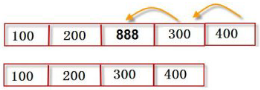• list中相邻元素的地址：对于列表a = [10, 20, 30, 40]来说，list里面的物理地址是连续的。
a = [1,2,3]
print(id(a))
print(id(a))
输出结果：
1688590512
1688590528   #12+16   每个unicode是16位。

• 列表和字符串很多函数用法是相通的
• 不要一边遍历，一遍修改/删除，可以先挑出有用的元素

### 元组

• 不可变序列
• 元组的访问和处理速度比列表快。
• 与整数和字符串一样，元组可以作为字典的键，列表则永远不能作为字典的键使用
• Python的元组与列表类似，不同之处在于元组的元素不能修改

• 索引访问
• 切片操作
• 连接操作
• 成员关系操作
• 比较运算操作
• 计数：元组长度 len()、最大值 max()、最小值 min()、求和 sum()等
• 排序 —— 返回一个list
>>> a = (20,10,30,9,8)
>>> print(sorted(a))
[8, 9, 10, 20, 30]
• 元组没有增加元素、修改元素、删除元素相关的方法

• a = (10,20,30)
• a = 10,20,30
• b = tuple()
• b = tuple("abc")
• b = tuple(range(3))
• b = tuple([2,3,4])

zip  zip(列表 1，列表 2，...)将多个列表对应位置的元素组合成为元组，并返回这个 zip 对象。
>>> a = [10,20,30] >>> b = [40,50,60] >>> c = [70,80,90] >>> d = zip(a,b,c)
>>> print(list(d),type(d))
[(10, 40, 70), (20, 50, 80), (30, 60, 90)]  <class 'zip'>

• divmod函数，divmod(13, 3)返回的也是一个元组(4, 1)，前面一个元素是商，后面一个是余数
• 当元组中只有一个元素时，比如tuple_a=(1, )必须要在元素后面加上逗号，不然会有歧义，比如如果不这样规定， 你无法区分(1)到底是元组还是一个数加上括号。
• 元组没有推导式
>>> (x for x in range(1,100) if x%9==0)
<generator object <genexpr> at 0x0000000002BD3048>

我们发现提示的是“一个生成器对象”。显然，元组是没有推导式的。 一个生成器只能运行一次。第一次迭代可以得到数据，第二次迭代发现数据已经没有了。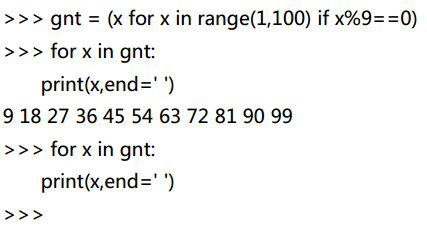### 字典

(1)  花括号 {}，不是list的[]；
(2)  键必须是唯一的；
(3)  键只能是简单对象，比如字符串、整数、浮点数、bool值。

• 重新赋值：score[‘虚竹’] = 91；
• 增加元素：score[‘慕容复’] = 88；
• 删除元素：del score[‘萧峰’]；
• 建立一个空字典：d = {}
• 字典推导式：{key_expression : value_expression for 表达式 in 可迭代对象}
统计文本中字符出现的次数：
>>> my_text = ' i love you, i love sxt, i love gaoqi'
>>> char_count = {c:my_text.count(c) for c in my_text}
>>> char_count
{' ': 9, 'i': 4, 'l': 3, 'o': 5, 'v': 3, 'e': 3, 'y': 1, 'u': 1, ',': 2, 's': 1, 'x': 1, 't': 1, 'g': 1, 'a': 1, 'q': 1}

# 方法1. 创建有数据的字典  (比较常用)
r1 = dict(name = "高小一",age = 18,salary = 30000,city = "北京")
print(r1,type(r1))
# 输出结果 {'name': '高小一', 'age': 18, 'salary': 30000, 'city': '北京'} <class 'dict'>

#  方法2. 用zip函数来创建字典对象
k = ["name", "age","job"]
v = ["gaoqi", 18, "techer"]
d = dict(zip(k,v))
print(d)
# 输出结果 {'name': 'gaoqi', 'age': 18, 'job': 'techer'}

#  方法3.  用fromkeys创建值为空的字典
a = dict.fromkeys(["name","age","job"])
print(a)
# 输出结果 {'name': None, 'age': None, 'job': None}

# example of using dict
score = { "萧峰": 95, "段誉": 97, "虚竹": 89 }
print(score.get("段誉"))    # 推荐使用，如果找不到的话，返回None
print(score["萧峰"])        # 如果找不到的话，会报错

dict = {'Name': 'Runoob', 'Age': 7}
for i,j in dict.items():    #分别对应字典中的键和值
print(i, ":\t", j)

#输出结果
Name :   Runoob
Age :    7

d = {'Name': 'gaoqi', 'Age': 18,"address":"西三旗001号楼" }
for  x in d:  #  遍历字典所有的key
print(x)

for  x in d.keys():  # 遍历字典所有的key
print(x)

for  x in d.values():  #  遍历字典所有的value
print(x)

for  x in d.items():  #   遍历字典所有的"键值对"
print(x)

#  第一个结果
Name
Age

#  第二个结果
Name
Age

#  第三个结果
gaoqi
18

#  第四个结果
('Name', 'gaoqi')
('Age', 18)
('address', '西三旗001号楼')

### 集合

• 创建集合  a = {3,5,7}
• 将列表/元组转为集合  a = ['a','b','c','b']    b = set(a)
• 集合操作：
• 并集  a|b
• 交集 a&b
• 差集 a.difference(b)     # a中删除和b中重复的元素
• 集合推导式：和列表推导式很类似
>>>  {x for x in range(1,100) if x%9==0}
{99, 36, 72, 9, 45, 81, 18, 54, 90, 27, 63}

## 变量的引用和拷贝

### 变量小知识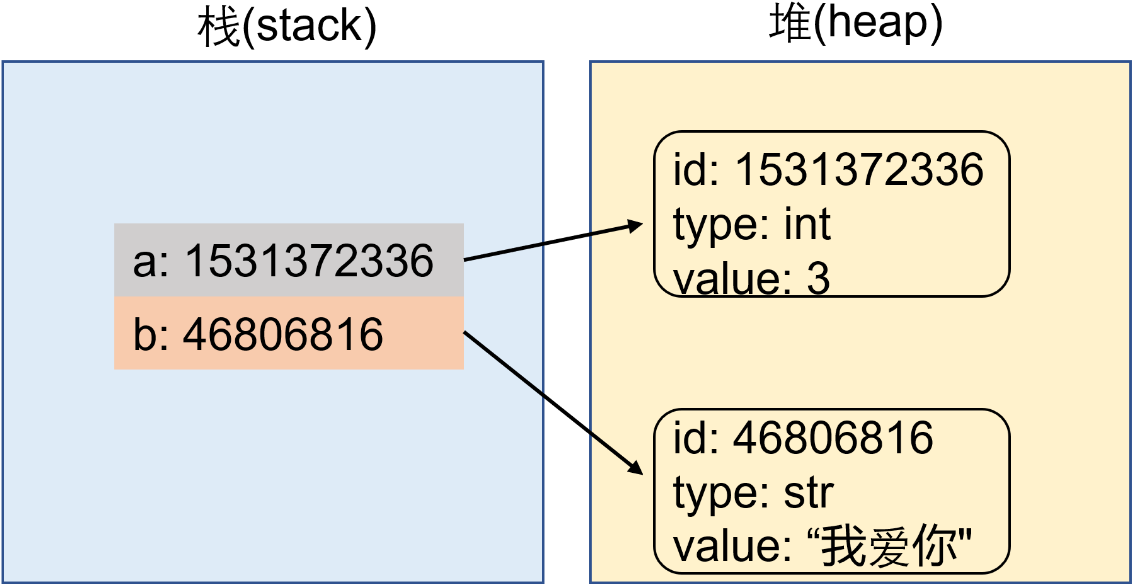• 如图是将3赋值给a的过程：a=3，以及将"我爱你"赋给变量b的过程。变量是对象的引用，因为变量存储的是对象的地址，变量通过这个地址来引用对象。变量位于栈内存，对象位于堆内存。
• del a 删除a其实是删除栈，而3这个对象没有变量取引用，就会被垃圾回收器回收，清空内存空间。
• a=b=3  等价于a=3然后b=3，二者的物理地址一样。
• a,b,c=4,5,6 分别赋值。 a, b = b, a表示a和b的值互换。
• float(3) 是生成一个新的对象，而不是修改原来的对象，int(), round()也是一样。
• a=3
a= a+1也是生成一个新的对象
• int(3.94)和round(3.94)结果的区别？

### 浅拷贝和深拷贝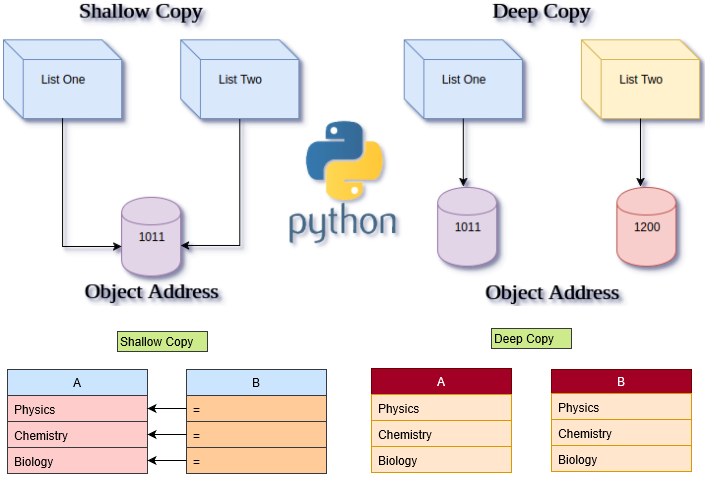Ashallow copy is one which makes a new object stores the reference of another object. While, in deep copy, a new object stores the copy of all references of another object making it another list separate from the original one.

Thus, when you make a change to the deep copy of a list, the old list doesn't get affected and vice-versa. But shallow copying causes changes in both the new as well as in the old list. This copy method is applicable in compound objects such as a list containing another list. 参见下面的例子：

import copy

a = [ [1, 2, 3], [4, 5, 6] ]
b = copy.copy(a)

c = [ [7, 8, 9], [10, 11, 12] ]
d = copy.deepcopy(c)

print(a)
print(b)

a = 23
b = 98

print(a)
print(b)

print("\n")

print(c)
print(d)

c = 23
d = 98

print(c)
print(d)

""" 输出
[[1, 2, 3], [4, 5, 6]]
[[1, 2, 3], [4, 5, 6]]
[[98, 2, 3], [4, 5, 23]]
[[98, 2, 3], [4, 5, 23]]

[[7, 8, 9], [10, 11, 12]]
[[7, 8, 9], [10, 11, 12]]
[[7, 8, 9], [10, 11, 23]]
[[98, 8, 9], [10, 11, 12]]
"""

### 函数中参数的传递

• 【可变对象】进行写操作：直接作用于原对象本身；
• 【不可变对象】进行写操作：会产生一个新的对象空间，并用新的值填充这块空间；
• 传递不可变对象时，不可变对象里面包含的子对象是可变的。则方法内修改了这个可变对象，源对象也发生了变化；
• 可变对象：列表、字符串、字典、集合和自定义的对象等；
• 不可变对象：数字、字符串、元组、function等；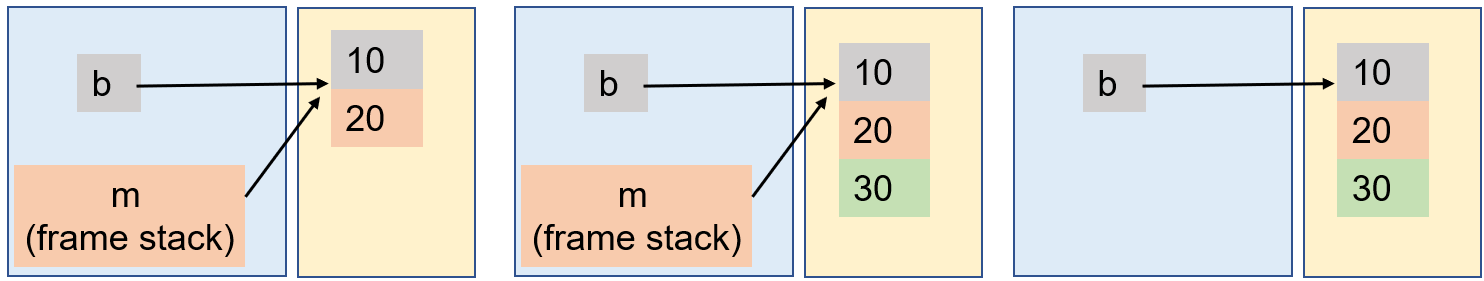b = [10, 20]
def f2(m):
print("m:",id(m))  # b和 m是同一个对象
m.append(30)     # 由于m是不可变对象，不创建对象的拷贝，直接修改这个对象

f2(b)
print("b:",id(b))
print(b)  # 输出 [10, 20, 30]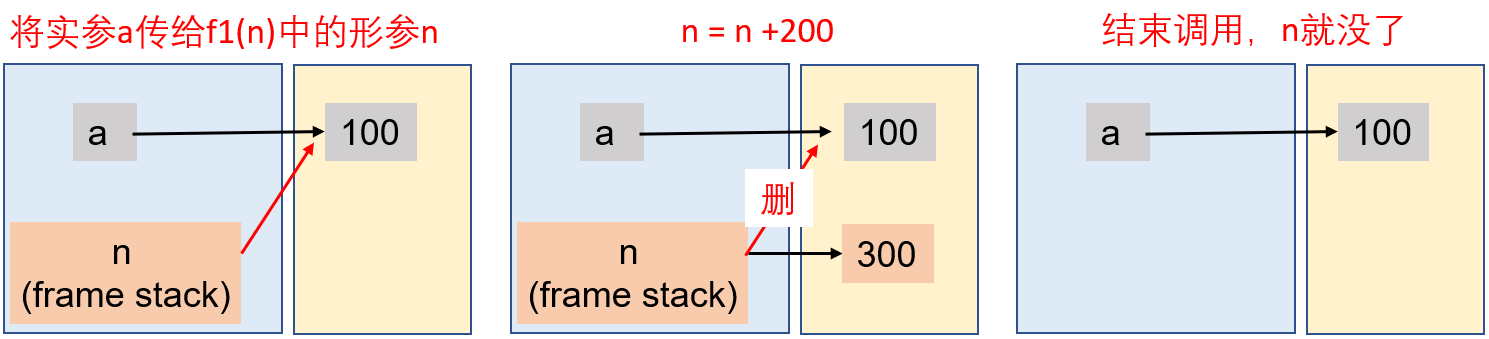a = 100
def f1(n):
print("n:",id(n))  # 传递进来的是a对象的地址
n = n+200       # 由于a是不可变对象，因此创建新的对象n
print("n:",id(n))  # n 变成新的对象
print(n)

f1(a)
print("a:",id(a))
#print(n)   # 这句会报错，因为函数中引入的 n是stack frame，用完就被垃圾回收了


x

x

101. 函数中的参数

1. *param（一个星号），将多个参数收集到一个“元组”对象中。
2. **param（两个星号），将多个参数收集到一个“字典”对象中。

def f3(a,b,*c,**d):
print(a,b,c,d)

f3(8,9,20,30,name='gaoqi',age=18)

8 9 (20, 30) {'name': 'gaoqi', 'age': 18}

102. eval()函数

## 程序结构

### if 语句

if.....elif.....elif........else 多分支选择结构，最后一个else是可选的，这其实就是C语言中的switch语句(python中没有switch)，之前我们在arduino用到过，也类似labview中的条件结构。一旦满足某个条件，就跳出整个大if。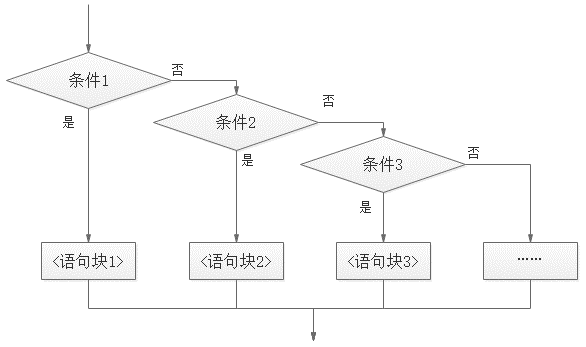• 赋值符不能出现在条件表达式中，比如if 3<c and (c=20):
•  if......if.......if....... 是顺序结构，不是多分支选择结构，需要把每一个if都执行一遍。

### for循环

# this shows how to jump out of a loop
for i in range(10):
a = input()
if a == "EOF":
break

# this shows how to skip one loop and do the rest loop stuff
for score in data[1:]:
point = int(score)
if point < 60:
continue
sum += point

• break 语句：在while 循环或者for.... in 循环，想提前跳出循环，可以用if....break语句，其中if给出跳出的限定条件，特别注意对齐方式；
• continue语句break是彻底地跳出循环，而continue只是略过本次循环的余下内容，直接进入下一次循环，多个循环嵌套时，continue 也是应用于最近的一层循环。(上面代码中低于60分的不计入总成绩)；
• 无论是continue还是break，其改变的仅仅是当前所处的最内层循环的运行，如果外层还有循环，并不会因此略过或跳出。

### else 语句

while/for......break......else...... ，当执行了break之后else的语句就不会执行了。

【操作】员工一共 4 人。录入这 4 位员工的薪资。全部录入后，打印提示“您已经全部录入 4 名员工的薪资”。最后，打印输出录入的薪资和平均薪资

salarySum= 0
salarys = []

for i in range(4):
s = input("请输入一共 4 名员工的薪资（按 Q 或 q 中途结束）")

if s.upper() == 'Q':
print("录入完成，退出")  # 给出中途退出的机制
break
if float(s) < 0:
continue

salarys.append(float(s))
salarySum += float(s)

else:
print("您已经全部录入 4 名员工的薪资")

print("录入薪资：",salarys)
print("平均薪资{0}".format(salarySum/4))


(1) Python for...else... 语句

### 其他

• 尽量减少循环内部不必要的计算；
• 嵌套循环中，尽量减少内层循环的计算，尽可能向外提；
• 局部变量查询较快，尽量使用局部变量。
#循环代码优化测试
import time

start = time.time()
for i in range(1000):
result = []
for m in range(10000):
result.append(i*1000+m*100)

end = time.time()
print("耗时：{0}".format((end-start)))

start2 = time.time()
for i in range(1000):
result = []
c = i*1000
for m in range(10000):
result.append(c+m*100)

end2 = time.time()
print("耗时：{0}".format((end2-start2)))


## 函数

python的函数分为四类：

• 内置函数(str()、int()、len()、input()、range()等
• 标准库函数(海龟模块、math模块等，需要import.obj导入)
• 第三方库函数
• 用户自定义函数

Python 中，一切都是对象。实际上，执行 def 定义函数后，系统就创建了相应的函数对象，也就是有栈和堆。函数对象后面的 () 就是调用的意思。

"""自定义函数格式说明

def function_name(paramters):
"""文档字符串"""    #解释说明这个函数的作用，大概说一下功能的实现方式
函数体/若干语句
"""

# example_1
def sayHello():
print ('hello world!')

sayHello()    # test

# example_2
def printMax(a,b):
"""实现两个数的比较，并返回较大的值"""
if a > b:
print(a,"larger value")
else:
print(b, "larger value")

printMax(10, 20)    #test

### 函数的参数

example_2里面的a、b是形参(formal parameter)，而10、20是实参(actual parameter)。下面介绍几种参数的形式：

• 没有参数：即example_1中的形式，调用函数，直接sayHello()即可；
• 有参数
• 没有默认值

def sayHello(name):
print ('hello ' + name)

sayHello("jacky")   # 输出hello jacky

• 有默认值

def sayHello(name = "jacky"):
print ('hello ' + name)

sayHello("Tom")     # hello Tom
sayHello()               # hello jacky
注：定义参数默认值的函数可以在调用时更加简洁，大量 Python 模块中的方法都运用了这一方式，让使用者在调用时可以提供尽可能少的参数。
有默认值的形参放在无默认值形参的后面

• 如果你想给部分参数提供默认参数，那么这些参数必须在末尾。比如 def func(a, b=5): 而不是def func(a=5, b):
• 输入参数不足的时候，匹配能匹配的部分，其他的输出默认值

def func(arg1=1, arg2=2, arg3=3):
print (arg1, arg2, arg3)

func(2, 3, 4)  # 输出 2 3 4
func(5, 6)      # 输出 5 6 3
func(7)          # 输出 7 2 3

func(arg2=8)                   # 指定参数值，输出  1 8 3
func(arg3=9, arg1=10)    # 指定参数值，输出10 2 9
func(arg1=13, 14)           # 指定参数，错误用法，指定的要放后面
func(15, arg1=16)           # 指定参数，错误用法，不能指定同一个

# 利用元组传递参数
def calSum(*args):
sum = 0
for i in args:
sum += i
print(sum)

calSum(1,2,3)   #输出 6
calSum(123,456) #输出 579
calSum()        #输出 0

# 利用字典传递参数
def printAll(**kargs):
for k in kargs:
print(k, ":",kargs[k])  # k表示key, kargs[k]表示key对应的value

printAll(x=4, y=5)
# 输出
"""
x : 4
y : 5
"""

tuple传递参数：

• 在变量前加上星号前缀*
• 调用时的参数会存储在一个 tuple（元组）对象中，赋值给形参；
• tuple 是有序的，所以 args 中元素的顺序受到赋值时的影响；

dict传递参数：

• 在变量前加上双星号前缀**
• func(**kargs) 则是把参数以键值对字典的形式传入；
• 字典是无序的，所以在输出的时候，并不一定按照提供参数的顺序；
• 同样在调用时，参数的顺序无所谓，只要对应合适的形参名就可以了；
• 不受参数数量、位置的限制；

def func(x, y=5,*a, **b):
print(x,y,a,b)

func(1)     # 1 5 () {}
func(1,2)   # 1 2 () {}
func(1,2,3)  # 1 2 (3,) {}
func(1,2,3,4)  # 1 2 (3, 4) {}
func(x=1)     # 1 5 () {}
func(x=1,y=1)  # 1 1 () {}
func(x=1,y=1,a=1)  # 1 1 () {'a': 1}
func(x=1,y=1,a=1,b=1)  # 1 1 () {'a': 1, 'b': 1}
func(1,y=1)    #  1 1 () {}
func(1,2,3,4,a=1)  # 1 2 (3, 4) {'a': 1}
func(1,2,3,4,k=1,t=2,o=3) # 1 2 (3, 4) {'k': 1, 't': 2, 'o': 3}


### 全局/局部变量-作用域-LEGB规则

• 在函数内部定义的变量；
• 不同的函数可以有相同的变量名，不会产生影响；
• 它的作用是临时保存函数中的数据，函数被执行完，该数据就没有了，每次调用该函数，就会形成一个新的栈帧(stack frame)，函数运行完一次，这种栈帧就被丢掉，类似胶卷一样，栈帧也是有栈和堆；
• 如果局部变量和全局变量同名，则在函数内隐藏全局变量，只使用同名的局部变量；
• 效率： 局部变量的查询和访问速度比全局变量快，优先考虑使用，尤其是在循环的时候。在特别强调效率的地方或者循环次数较多的地方，可以通过将全局变量转为局部变量提高运行速度，但是大部分情况下不需要关注效率。

• 在函数外部定义的变量；
• 全局变量的使用降低了函数的可读性和通用性，尽量避免使用；
• 对于不可变类型的全局变量，要在函数内修改，需要使用global时修改全局变量；
• 对于可变类型的全局变量，不使用global时，也可以在函数内进行修改
• 注：
• 可变对象：列表、字符串、字典、集合和自定义的对象等；
• 不可变对象：数字、字符串、元组、function 等；

# example_1 变量x的值在函数内容发生改变，但是并不传递到函数外
def func(x):
print("x in the beginning of func(x): ", x)
x = 2
print("x in the end of func(x): ", x)

x = 50
func(x)
print("x after calling func(x): ", x)

# 输出
"""
x in the beginning of func(x):  50
x in the end of func(x):  2
x after calling func(x):  50
"""

# example_2 函数内部定义了全局变量，于是将赋值后的x传递到函数之外
def func():
global x
print("x in the beginning of func(x): ", x)
x = 2
print("x in the end of func(x): ", x)

x = 50
func()
print("x after calling func(x): ", x)

# 输出
"""
x in the beginning of func(x):  50
x in the end of func(x):  2
x after calling func(x):  50
"""

### 匿名函数

【匿名函数】(anonymous function)：在Python中，匿名函数是没有定义名称的函数。虽然def在Python中使用关键字定义了普通函数，但使用关键字定义了匿名函数lambda。因此，匿名函数也称为lambda函数，其特点如下：

• Lambda函数可以具有任意数量的参数，但只能有一个表达式，表达式被求值并返回；
• lambda表达式能表达的逻辑很有限，能用lambda的地方其实都可以用def替代，只是前者更简洁，但是能用def的地方，不一定能用lambda；
• lambda表达式虽然简洁，但是它的主体只能是一个表达式，不可以是代码块，甚至不能是命令，比如不能使用print命令；
• lambda 表达式的语法格式： lambda 参数列表: 表达式
# 匿名函数lambda的使用示例
sum = lambda a,b,c: a+b+c
print(sum(1,2,3))  # 输出 6

# 等价于下面的有名函数
def sum(a,b,c):
return a+b+c

print(sum(1,2,3))

# lambda位于有名函数里面的例子
def fn(x):
return lambda y: x+y

a = fn(2)  # 得到 2+y
print(a(3))  # y=3, 于是2+3 =5,即输出5

# 关于lambda更复杂的例子
g = [lambda a:a*2,lambda b:b*3,lambda c:c*4]
print(g(6),g(7),g(8))  #输出 12 21 32


help(printMax)可以得到printMax函数注释说明的文字内容

### 返回值

def test():
print("abdc")
return  # 两个作用，返回值；结束函数执行
print("def") #在return之后，所以不被执行

test() # 只输出abd
• 两个作用：
• 执行并返回值
• 结束函数
• 如果函数体中不包含return语句，则返回None值
• 要返回多个返回值，使用列表、元组、字典、集合将多个值"存起来"即可

### 嵌套函数(内部函数)

• 封装 —— 数据隐藏，外部无法访问嵌套函数；
• 贯彻DRY(Don't Repeat Yourself)原则，嵌套函数可以让我们在函数内部避免重复代码；
• 闭包 —— 后面会详细解释
# 嵌套函数的定义
def outer():
print("outer running")

def inner01():
print("inner01 running")

inner01()  # 在outer里面调用inner

outer()
# 输出outer running
# inner01 running

# 例子-使用嵌套函数避免重复代码

# 修改前
def printChineseName(name,familyName):
print("{0} {1}".format(familyName, name))

def printEnglishName(name,familyName):
print("{0} {1}".format(name, familyName))

# 修改后——使用 1 个函数代替上面的两个函数
def printName(isChinese,name,familyName):
def inner_print(a, b):
print("{0} {1}".format(a, b))

if isChinese:
inner_print(familyName, name)
else:
inner_print(name, familyName)

printName(True,"小七","高")  # 输出 高 小七
printName(False,"George","Bush") # 输出 George Bush


xx

## 注释和Debug

### 注释

• 一般不要在代码里面写中文注释，如果非要写，请在第一行输入# coding: gbk
• Ctrl+/  注释某一行，三个键一起，再作用一次就接触注释
• 注释某一段：使用三个单/双引号。选中内容，然后按住Shift不放，然后连续敲击三次引号键。参见这里
# 注释功能
"""
这是我的python第一课
我学习了基本语法和常用运算符
"""

# 换行输出功能
print("""这是我的python第一课
我学习了基本语法和常用运算符"""
)


### 排错和调试

• python shell
三个右括号 >>> 是 python 输入的提示符，它表示 python 解释器已经准备好了，等待你的命令。
python shell 可以非常方便的运行 python 语句，这一点对调试、快速组建和测试相当有用。当你在编写代码的过程中，对一些方法不确定的时候，可以通过 python shell 来进行试验。
• 查询
• 某个对象的物理地址：id(变量名/obj)
• 查询对象的类: type(obj)
• 输出obj中储存的数据：print(obj)。
• debug方法1 —— 选中某一行(左侧出现红点) 然后右键debug，接着可以使用step over/step into 来陆续执行每一行代码，另外还有step out(三者区别见这里)。如果我们选中两行，也就是左侧设定两个红点thread，debug的时候先执行到第一个红点处，然后我们点“run to cursor”就会直接从第一个红点处执行到第二个红点处，参见视频
• debug方法2 —— 每生成一次变量，让这个变量输出一次，看程序循环到哪里就循环不动了。下面分别给出原程序和改造之后的程序(输出调试信息)

# original one
import random
a =0
for i in range(5):
b = random.choice(range(5))
a +=i / b
print(a)

# revised one
import random
a = 0
for i in range(5):
print("i: %d" % i)
b = random.choice(range(5))
print("b: %d" % b)
a +=i / b
print("a: %d" % a)
print()
print(a)

• 调出帮助文档：直接在pycharm界面按F1的话，出现的是pycharm网页的帮助文档。选中自己编写的某个代码使用的函数，选鼠标选中后，左手按住Ctrl，右手单击一下；或者先选中后，然后按shift+F1。
• 异常处理( try…except )
除去开发者可以避免的错误，使用者可能输入一些非法值，这个时候就必须给出响应的“反馈”，常用的是try....except语句，比如打开一个本身就不存在的文件：文件存在，被打开，给出“回应”；文件不存在，同样要给出“回应”。总之，程序员的程序给用户用，必须考虑到所有情况。
try:
f = open("non-exist.txt")
print("File opened!")
f.close()
except:
print("File not exists.")
print("Done")


## 文件操作和输出格式

### 文件操作

f = open('任缥缈.txt', encoding= 'utf-8')   #中文的编码 ，打开文件
print(s)        # 输出内容
f.close()      # 关闭文件

# write
f = open(‘write_test.txt’, mode = ‘w’, encoding = ‘utf-8’)  # w表示写入， 生成新文件
f.write(‘我很好\n’)
f.write(‘你好吗\n’)
f.close()

### 输出

\ 被称作【转义字符】，用处如下：

• 换行  \n
• 横向制表符  \t 表示字符串中的制表符（相当于按一下tab键的效果）
• 续行符   \(在行尾时)
print("tiantian\
xiangshang")
#输出 tiantian xiangshang (同一行)
• 反斜杠符号  \\
• 单引号   \'
• 退格 \b
• 字符串里面有引号，那么在每个引号之前加上\，程序就可以区分不同引号(a = (' He said, \"I\'m yours!\" ' ))

\t 被称作制表符(Box Drawing)

% 对字符串进行格式化，其中%d 替换整数，%f 替换小数，%.2f替换保留两位小数，%s 替换字符串。例子：

• print('My age is %d' % 18)
• print('Price is %f' % 4.99)
• name = 'Crossin'
print('%s is a good teacher.' % name)
• print("%s's score is %d" % ('Mike', 87))，一个字符串里面替换两个，这里的('Mike', 87)就是元组
• 数字字符串化 print('My age is ' + str(18))，或者print("My age is", str(18))

format 函数format函数是用来替代前面的%的，它可以接受不限个数的参数，而且位置可以不按顺序。

# format指定顺序
a = "我叫{0}，我今年{1}岁了，我的专业是{2}。"
print(a.format("呼哨","25","材料科学与工程"))

# format不指定顺序
a = "我叫{name}，我今年{age}岁了，我的专业是{major}。老师说{name}成绩一般。"
print(a.format(major= "材料科学与工程", name="呼哨", age=18))

# format数字格式化
a = "我是{0}，我的存款有{1:.2f}"
print(a.format("高淇",3888.234342))
#输出：我是高淇，我的存款有3888.23


format填充与对齐(排版)

^、<、>分别是居中、左对齐、右对齐，后面带宽度
:号后面带填充的字符，只能是一个字符，不指定的话默认是用空格填充

"{:*>8}".format("245")

{:*>8}表示右对齐，8表示占据8个位置，由于我们只有一个三位数，所以左侧的5个位置由我们制定的*来占据。

"我是{0},我喜欢数字{1:*^8}".format("高淇","666")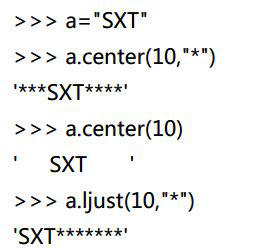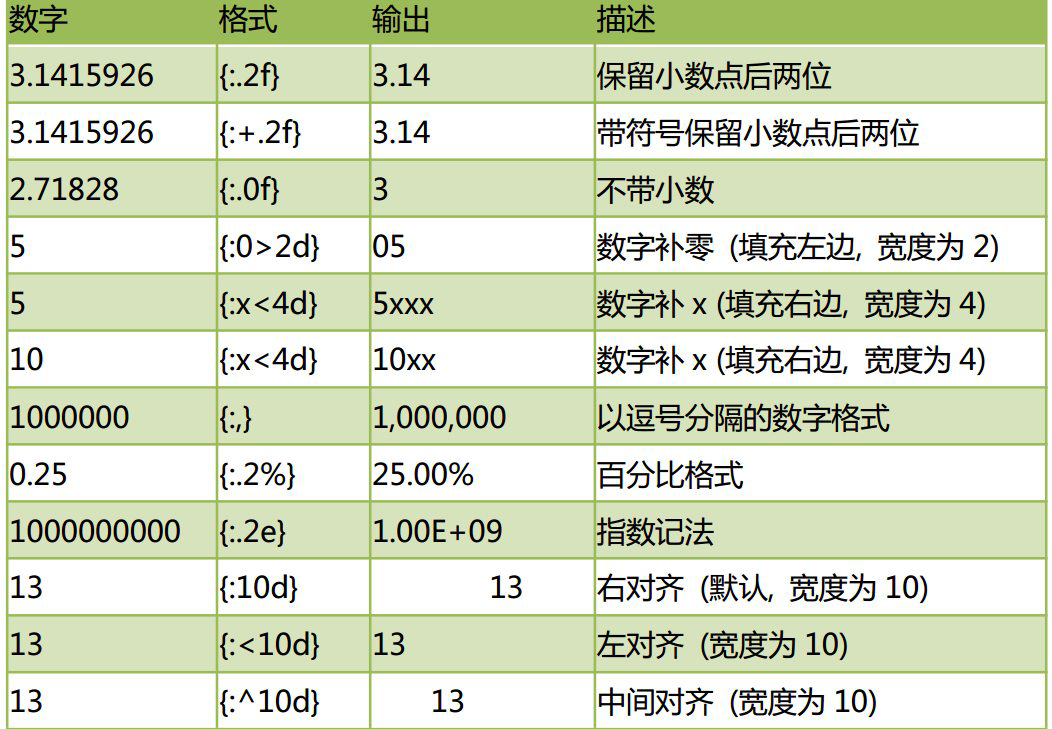• 不换行打印
end=' '   输出一个之后，空格，输出第二个。end 参数的作用是指定 print 结束之后的字符，默认是回车。你可以试试设置成不同字符的效果。
• 输出一个变量的数据类型
比如a = 1，然后print(a, type(a))得到的是1 <class 'int'>，这里的int可以变为字符串str、bool类型等。
• 错误的print方式
print('Hello'+1) 或者print('hello%d' % '123')
前者的错误原因：加号只能用于数字之间或者字符串之间；%d对应的是数字，而不是字符串，加上引号的数字就变成了字符串。

## 其他

xx

xx

### cd命令

• 在terminal输入python 并敲下回车，就可以进入python环境了；
• C:\Users\hujie>dir   输入dir得到当前文件夹下所有子文件的信息；
• 输入cd 得到目录名；
• 输入cd.. 返回到上一级目录；
• 输入cd  目录名， 进入当前目录的子目录。
注：dir-directory(目录)；cd-change directory(改变目录)

### python 2和3

• print('this is version 3') , 而2中不需要括号(3中print是一个函数，所以有括号)；
• python 2和3的不换行输出
python 2：print '*',
python 3：print('*', end=' ')    # python每次print默认以换行结束，这里使用end，将换行结束变成了空格结束。
• 后续碰到了再补上，学习别人的程序，要搞清楚是2还是3。

### name变量

python代码既可以作为一个脚本被执行，也可以作为一个模块被引入(相当于定义的函数)，有时候我们只想在它作为一个脚本被执行的时候执行一些代码，这就需要判断 __name__ 变量(前后各有两个下划线)的值。作为python的内置变量，它是每个python模块必备的属性，但是它的值取决于你是如何执行这段代码的。

if __name__ == '__main__':  可以判断代码是作为脚本被执行还是作为模块被引入：

• 当你直接执行一段脚本的时候，这段脚本的 __name__ 变量等于 __main__ ，当这段脚本被导入其他程序的时候， __name__ 变量等于脚本本身的名字；
• 在hello.py里面写入print(__name__)，当它被直接执行时，输出的是__main__；但是在Python Console引入该py文件，即import hello得到的是hello，而不是__main__。

## 模块

### random模块

import random就是导入random模块，但是这个模块里面有很多种类的函数，我们必须选择需要的进行调用，比如random.randint(1, 10) 和random.choice([1, 3, 5])。

• 用法举例： 比如 from random import randint(引入随机数模块)，接下来你就可以用randint来产生随机数，比如num = randint(1, 100)
• random.randint(a, b)可以生成一个a到b间的随机整数，包括a和b。注：a、b必须都是整数。
• random.randint(3, 3) 结果只有3。
• random.random() 生成一个随机浮点数[0.0, 1.0)左边闭合，右边开。
• random.uniform(a, b)生成a、b之间的随机浮点数。不过与randint不同的是，a、b无需是整数，也不用考虑大小。
• random.choice(seq)
• random.choice([1, 2, 3, 4, 5, 7, 13])   #list
• random.choice("hello")          #字符串
• random.choice(["hello", "world"])   #字符串组成的list
• random.choice((1, 2, 3))    #元组
• random.randrange(start, stop, step)
• random.sample(population, k) 从population序列(list、元组、字符串)中，随机获取k个元素，生成一个新序列。sample不改变原来序列。
• random.shuffle(x)  把序列x中的元素顺序打乱。shuffle直接改变原有的序列

### math模块

• math.pi # 圆周率π
• math.e # 自然常数
• math.ceil(x) # 对x向上取整
• math.floor(x) # 对x向下取整
• math.pow(x,y) # 指数运算
• math.log(x) # 对数，默认基底为e
• math.sqrt(x) # 平方根
• math.fabs(x) # 绝对值
• math.sin(x)
• math.degrees(x) # 弧度转角度

### time模块

unix时间戳计算机领域的特殊事件epoch，它表示的时间是1970-01-01 00:00:00 UTC
time.time()返回的就是从epoch到当前的秒数（不考虑闰秒），这个值被称为unix时间戳。 对比labview的起始时间是1904年1月1日8点。

import time
starttime = time.time()
print ('start:\%f' \% starttime)
for i in range(10):
print (i)
endtime = time.time()
print ('end:\%f' \% endtime)
print ('total time:\%f' \% (endtime-starttime))

time.sleep(secs) 让程序暂停secs秒，类似于labview中的wait或者arduino中的delay。比如在抓取网页的时候，适当让程序sleep一下，可以减少短时间内的请求，提高请求的成功率。

## 常见函数

### eval 函数

• 将字符串 str 当成有效的表达式来求值并返回计算结果
• 比如，从客户端发来一段代码，或者从某个文件里读取的一段代码，可以通过eval函数执行

# 例子-1
s = "print('abcde')"
eval(s)

# 例子-2
a = 10
b = 20
c = eval("a+b")
print(c)

# 例子-3
dict1 = dict(a=100,b=200)
d = eval("a+b",dict1)
print(d)


xxx

(1) 高阶函数—廖雪峰
(2) 一文搞懂python的map、reduce函数—知乎

### reverse函数

a=[1,2,3,4]
b=a.reverse()
print(b)   # 返回None
print(a)   #返回[4, 3, 2, 1]

a=[1,2,3,4]
a.reverse()
print(a)

a=[1,2,3,4]
print(id(a))   # 输出33651448
a.reverse()
print(id(a))  # 地址不变 输出33651448

b=reversed(a)
b = list(b)
print(b)   # [1, 2, 3, 4]
print(b)   # [1, 2, 3, 4]

b=reversed(a)    # 返回迭代器，指针不断移动
print(list(b))  # [1, 2, 3, 4]
print(list(b))  # []  因为第一次已经遍历完了


reverse和之前讨论的sort类似。

### enumerate函数

aa=["a","b","c","f","a"]
print(enumerate(aa))   # 输出 <enumerate object at 0x01C066C0>
print(list(enumerate(aa))) # 输出[(0, 'a'), (1, 'b'), (2, 'c'), (3, 'f'), (4, 'a')]

# 用枚举
aa = ['a', 'b', 'c', 'f', 'a']
for index,s in enumerate(aa):
if s== "a":
print(index)

# 如果用老方法
aa = ['a', 'b', 'c', 'f', 'a']
bb = 0
for x in aa:
if x == "a":
print(bb)
bb += 1


### map函数

map()是python的内置函数，会根据提供的函数对指定序列(如列表、元组等)做映射并通过把函数f依次作用在序列的每个元素上，得到一个新的list并返回。map()函数不改变原有的序列，而是返回一个新的序列。

###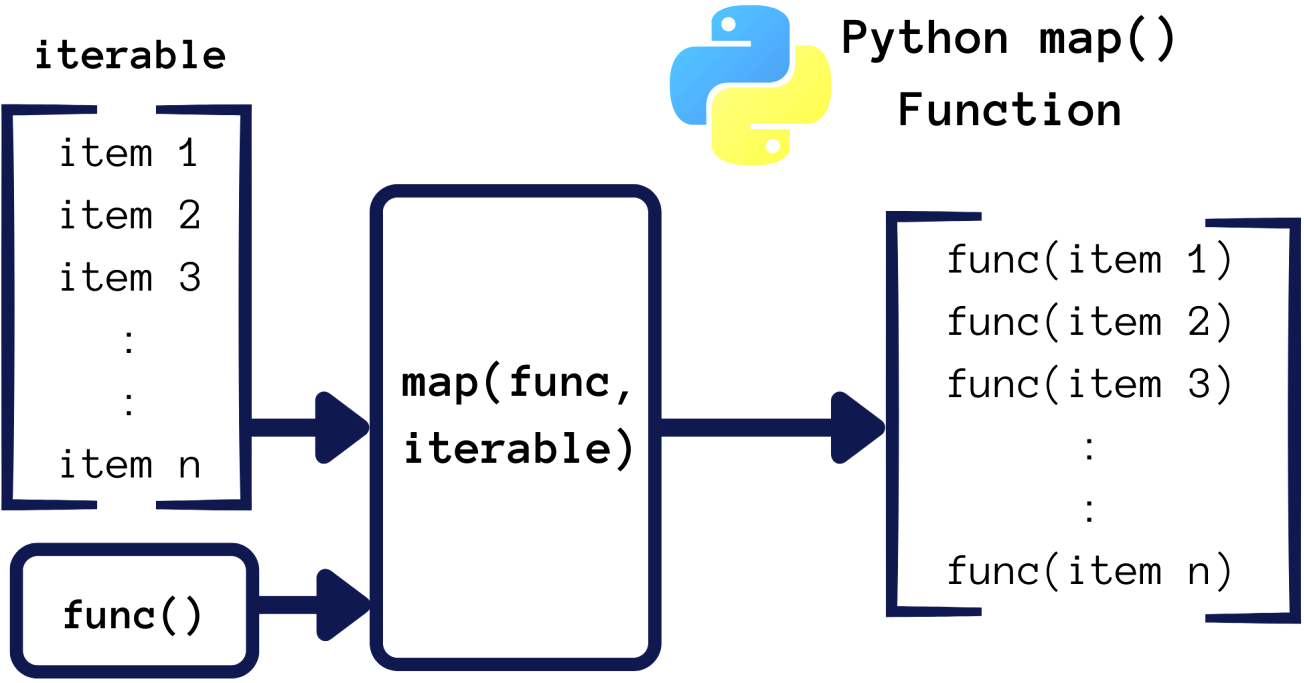# example_1 将list中的字符型的数字，转换成int
a = list("1213456789")
print(a)   # ['1', '2', '1', '3', '4', '5', '6', '7', '8', '9']
b = map(int, a)
print(b)  # <map object at 0x018EF8B0>
print(type(b)) # <class 'map'>
print(list(b)) # [1, 2, 1, 3, 4, 5, 6, 7, 8, 9]

#  example_2 将数字的list中的每个数字都平方一下
a = [1, 2, 3, 4, 5, 6, 7, 8, 9]
def f(x):
return x*x
b = map(f, a)
print(list(b))  # [1, 4, 9, 16, 25, 36, 49, 64, 81]

#  example_3 两个或者多个list的情况
a = map(lambda x,y:x**y,[1,2,3],[1,2,3])
print(a)   # <map object at 0x01805E70>
print(list(a)) # [1, 4, 27]

# example_4 func不仅只接收函数，同样可接收lambda表达式
a = [1, 2, 3, 4]
b = map(lambda x: x*x, a)
print(list(b))  #  [1, 4, 9, 16]

x

### reduce 函数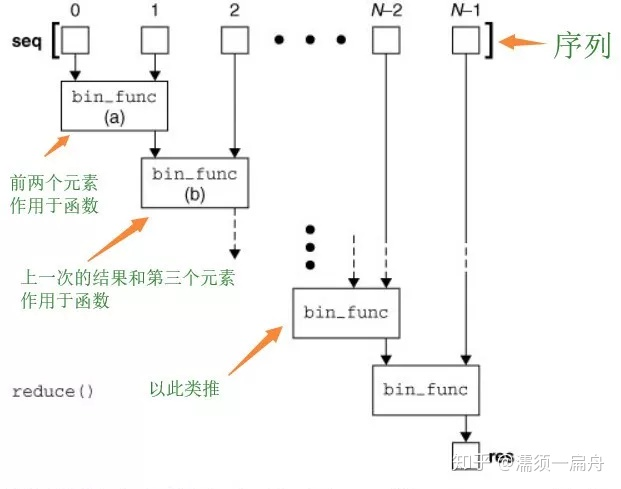reduce的工作过程是 ：在迭代序列的过程中，首先把 前两个元素（只能两个）传给 函数，函数加工后，然后把 得到的结果和第三个元素 作为两个参数传给函数参数， 函数加工后得到的结果又和第四个元素 作为两个参数传给函数参数，依次类推。

# 计算加和
from functools import reduce   # reduce函数不能直接使用，必须先import
def add(x, y) :            # 两数相加
return x + y
a = [1,2,3,4,5]
print(sum1)
print(sum2)      # 计算列表和：1+2+3+4+5+10

#计算阶乘，两种方法
from functools import reduce
n = 5
print(reduce(lambda x, y: x*y,range(1, n+1))) # 输出120

from functools import reduce
def f(x, y):
return x*y
n = 5
items = range (1, n+1)
factorial =  reduce(f, items)
print(factorial)   # 输出120


## 面向对象

36. 面向对象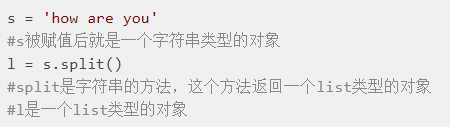print(dir(s))      得到字符串这种类的所有属性
print(dir(l))   得到list这种类的所有属性

77. 三元条件运算符(使代码简单、易读)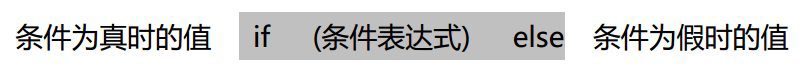num = input("请输入一个数字")
print( num if int(num)<10 else "数字太大")if num%2==0:sum_even += num
else:sum_odd += num

86. 字符串驻留机制和字符串比较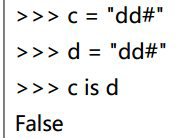我在pycharm中结果为True

(1)  ==,!=对字符串进行比较，是否含有相同的字符。

(2)  is / not is，判断两个对象是否同一个对象。比较的是对象的地址，即 id(obj1)是

89. 补充前面的多维列表

a = [
["高小一",18,30000,"北京"],
["高小二",19,20000,"上海"],
["高小一",20,10000,"深圳"],
]

1.

2.

92. 使用 zip()并行迭代

a = [10,20,30] b = [40,50,60] c = [70,80,90] d = zip(a,b,c)
print(d,type(d))

a = [10,20,30] b = [40,50,60] print(dict(zip(a,b)))

names = ("高淇","高老二","高老三","高老四")
ages = (18,16,20,25)
jobs = ("老师","程序员","公务员")
for name,age,job in zip(names,ages,jobs):
print("{0}--{1}--{2}".format(name,age,job))

names = ("高淇","高老二","高老三","高老四")
ages = (18,16,20,25)
jobs = ("老师","程序员","公务员")
for i in range(3):
print("{0}--{1}--{2}".format(names[i],ages[i],jobs[i]))

106.  nonlocal关键字

nonlocal 用来声明外层的局部变量。
global 用来声明全局变量。

def
b=10
def
nonlocal  b     #可以在内层函数对b进行修改
b=20

108. 面向对象初识

109. 数据的进化

1.   最简单数据，就是我们按计算器的那种数据，30,40，50.4 等这些数字。

2.    数组，将同类型的数据放在一起，比如 [20,30,40][“aa”,”bb”,”cc”]

3.  结构体将不同类型的数据放到一起，是 C 语言中的数据结构 。比如每个人的户口，包含各种信息：出生年月、户籍地址、身高、血型等等。

4.  对象

110. 类  (类名首字母大写，驼峰原则)

python中一切皆对象，类(饼干磨具)也是对象，只不过是产生对象的“对象”。

123是地址，传给了self。self.name就是刚才那个对象的name属性=外部传进来的数据(张三)。init是initial的缩写。

111. __init__构造方法和__new__方法

s1 = Student('张三', 80) 的执行顺序
(1)  __new__()方法: 用于创建对象，但我们一般无需重定义该方法
(2) __init__()方法：初始化创建好的对象，初始化指的是：“给实例属性赋值

1. id（identity 识别码）
2. type（对象类型）
3. value（对象的值）
(1) 属性（attribute）
(2) 方法（method）

_init__()的要点如下：
1. 名称固定，必须为：__init__()
2. 第一个参数固定，必须为：self。 self 指的就是刚刚创建好的实例对象。
3. 构造函数通常用来初始化实例对象的实例属性，如下代码就是初始化实例属性：name和 score。

112. 实例属性

self对应于123这个物理地址，s1这个变量也引用123这个物理地址。123对应的对象是从磨具Student来的，我们可以对123这个地址的对象增加新的属性；但是如果再次调用Student的的话(s2=Student("高淇", 18))，会从磨具中套出一个新的对象，物理地址不再是123。

113. 实例方法

1. 都是用来完成一个功能的语句块，本质一样。
2. 方法调用时，通过对象来调用。方法从属于特定实例对象，普通函数没有这个特点。
3. 直观上看，方法定义时需要传递 self，函数不需要。

1. dir(obj)可以获得对象的所有属性、方法
2. obj.__dict__ 对象的属性字典
3. pass 空语句
4. isinstance（对象,类型） 判断“对象”是不是“指定类型”

## 应用实例展示

### 查天气

requests库的作用：包括gzip压缩、字符编码、json的自动处理。如果用Python自带的urllib.request库，工作量就会增加很多。

import requests
req = requests.get("http://hao123.com")
print(req)
req.encoding = "utf8"
content = req.text
print(content)

get是requests库中的一个函数，将打开网址得到的变量存入到req，而print(req)返回的是一个数组，如果是200则说明网站访问请求成功。red.encoding='utf-8'表示的是编码方式，因为里面有中文，所以指定这种编码方式。req.txt是req内容变量的text属性，按照文本的内容赋值给content，然后输出content即得到网站的代码，其中包含html+css+javascrpit等语言。我们把content的内容复制到记事本，然后把后缀改成html，那么同样可以用浏览器打开。

while True:
city = input('请输入城市,回车退出:\n')
if not city:  # 如果输入回车，那么意味着city是空，not空的布尔就是真，于是执行break
break
req = requests.get('http://wthrcdn.etouch.cn/weather_mini?city=%s' % city)
print(req.text)